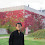### Function for Digital Unit Conversion in Power BI (KB, MB, GB...)

While displaying reports it is very common to convert the units. So today I will be displaying the function that can be used in order to convert the Units

Space =
VAR total =
SUM ( Table1[Used Space] ) + 0
RETURN
IF (
total < 1024,
FORMAT ( total, "#0.0# B" ),
IF (
total < POWER ( 220 ),
FORMAT ( total / POWER ( 210 ), "#0.0# KB" ),
IF (
total < POWER ( 230 ),
FORMAT ( total / POWER ( 220 ), "#0.0# MB" ),
FORMAT ( total / POWER ( 230 ), "#0.0# GB" )
)
)
)

So if you look into the function the core logic is

B --> KB then divide by POWER ( 210 )
B --> MB then divide by POWER ( 220 )
B --> GB then divide by POWER ( 230 )

And...

KB --> B then multiply by POWER ( 210 )
MB --> B then multiply by POWER ( 220 )
GB --> B then multiply by POWER ( 230 )

Use the above method in order to convert the units and display them in a friendly text

Happy Coding..!!

1.This is great! can you keep going to PB?

1.Sure, Thanks